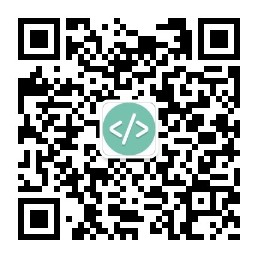# Objective-C块和变量

```NSArray *array = @[@25, @"abc", @7.2];

void (^print)(NSUInteger index) = ^(NSUInteger index)
{
NSLog(@"%@", array[index]);
};

for (int n = 0; n < 3; n++)
print(n);```

```NSArray *array;

void (^print)(NSUInteger index) = ^(NSUInteger index)
{
NSLog(@"%@", array[index]);
};

array = @[@25, @"abc", @7.2];

for (int n = 0; n < 3; n++)
print(n);```

```NSArray *array = @[@25, @"abc", @7.2];

void (^print)(NSUInteger index) = ^(NSUInteger index)
{
NSLog(@"%@", array[index]);
};

for (int n = 0; n < 3; n++)
print(n);```

__block示例：

```__block NSArray *array;

void (^print)(NSUInteger index) = ^(NSUInteger index)
{
NSLog(@"%@", array[index]);
};

array = @[@25, @"abc", @7.2];

for (int n = 0; n < 3; n++)
print(n);```### 相关软件推荐:

#### Objective-C编程

[美] Aaron Hillegass / 夏伟频、李骏 / 华中科技大学出版社 / 2012-9-25 / 58.00元

《Objective-C编程》讲述Objective-C编程语言和基本的iOS/Mac开发知识。作者首先从基本的编程概念讲起（变量、条件语句、循环结构等），接着用浅显易懂的语言讲解Objective-C和Foundation的知识，包括Objective-C的基本语法、 Foundation常用类 、内存管理、常用设计模式等，最后手把手教读者编写完整的、基于事件驱动的iOS/Mac应用。作者还穿插......一起来看看 《Objective-C编程》 这本书的介绍吧!